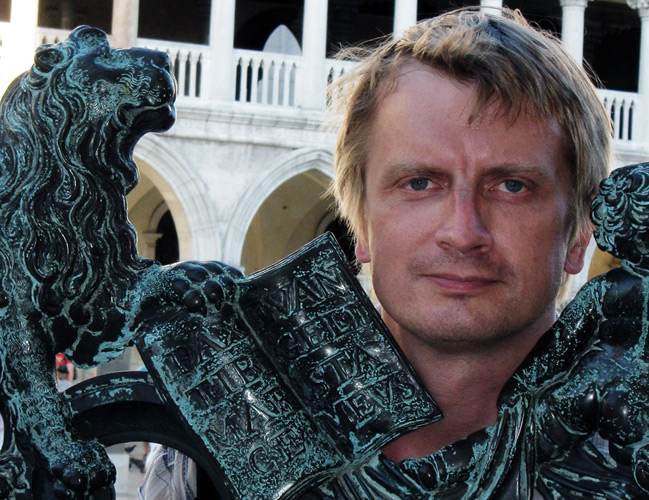my starting page Bogomolov's Lab. starting page     Alexey Gorodentsev's SHEAVES AND HOMOLOGICAL ALGEBRA Fall 2015 Contents: Program: PDF in English, PDF in Russian Recomended Textbooks Home Tasks Marking Rules and Exam

## Recomended Textbooks

• V.I.Danilov. Cohomology of Algebraic Manifolds. English version in: Algebraic Geometry II: Cohomology of Algebraic Varieties. Algebraic Surfaces. Encyclopaedia of Mathematical Sciences. Book 35. Springer (1995). Русская версия в книге «Алгебраическая геометрия–2». Итоги науки и техники. Совр. пробл. математ. Фунд. напр. Т. 35. Москва, ВИНИТИ, 1989, с. 5–130.
• P.Griffiths, J.Harris. Principles of Algebraic Geometry. (есть русский перевод)
• S.I.Gelfand, Yu.I.Manin. Methods of Homological Algebra. Part I. (есть русский оригинал)
• B.Iversen. Cohomology of Sheaves.
• C.A.Weibel. An Introduction to Homological Algebra.

Some of my own lecture notes (in Russian) are available here.
There is also an ntroduсtion to categories and fuctors (in English).

All problems marked with stars as well as all problems from the tasks numbered by non-integers are honorary. The maximal mark «10» can be achieved without solving them.
• Task 1: Categories and functors.
• Task 2: Adjoint functors and colimits (in Russian).
• Task 3: Complexes and homologies (in Russian).
• Task 3 1/2 (honorary): Exact categories (in Russian).
• Task 4: Exact couples and spectral sequencqs (in Russian).
• Task 5: Sheaves on topological spaces (in Russian).
• Task 6: Sections with compact support (in Russian).
• Task 7: Coherent sheaves (in Russian).

## Marking rules and Exam

Let H and E be the total amounts of problems you have solved among the problems given in the Home Tasks and in the Written Exam respectively (both are computed as percentage [total number of solved problems]:[total number of non-honorary problems] and may be >100 if you solved honorary problems). The final mark is computed as FIN MARK = MIN(140, H+E)/14. Thus, to get «10», it is enough to have H+E to be at least 140.

Final written examination takes place in Friday, December 25 from 1200 until 1630 in room 311. Exam tasks are here.